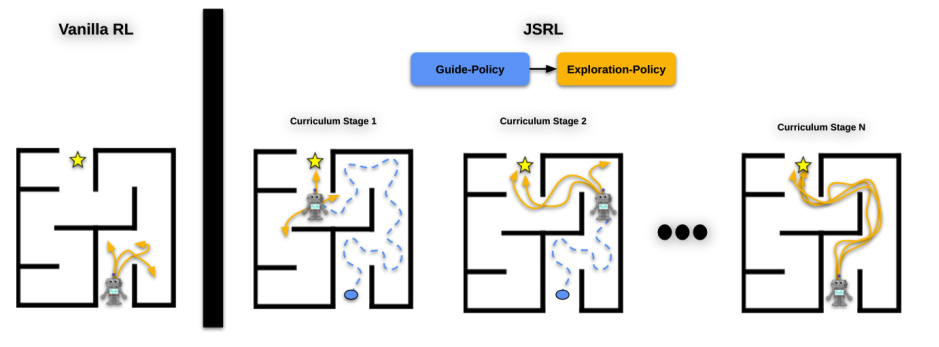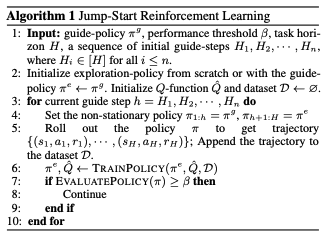# Catch up on the latest AI articles# Jump-Start RL: Streamlines Search By "guiding" With Pre-learned Strategies!

3 main points
✔️ Propose Jump-Start RL, a framework for efficient search using pre-trained measures
✔️ Analyze the dependence of pre-trained measures on performance through theoretical analysis
✔️ Experiments confirm results outperforming existing methods

writtenby
(Submitted on 5 Apr 2022 (v1), last revised 7 Jul 2023 (this version, v2))

Subjects: Machine Learning (cs.LG)code：The images used in this article are from the paper, the introductory slides, or were created based on them.

## Background

Reinforcement Learning (RL) is a framework for learning optimal behavioral strategies through repeated trial and error by interacting with the environment. However, it is known that a large number of interaction samples are required to learn the optimal strategy from scratch. This is especially true for problems that require large-scale search with large state and action spaces. To cope with such problems, methods to improve learning efficiency by using pre-trained policies and value functions have attracted much attention. The idea is to reduce the number of interactions required for learning by using "policies or value functions with some good performance" as initial values.

## Existing Research and Issues

### Imitation learning + RL

The method learns a policy $\pi$ by imitation learning from the data $D=\{s_i, a_i, r_i, s'_i\}_{i=1}^n$ of the interaction with the given environment, and then performs reinforcement learning using the learned policy $\pi$ as the initial policy. In order to use the value-based reinforcement learning method, not only the policy but also the value function of the learned policy is required as an initial value, and methods have been developed to deal with this problem by including not only the previously learned policy $\pi$ but also the data $D$ used for learning in the replay buffer.

### Offline Reinforcement Learning + RL

In order to improve the connection with value-based reinforcement learning methods, a method is also proposed to perform pre-training with off-line reinforcement learning, which estimates not only measures but also value functions from off-line data $D$.

In summary, we found that existing methods require the use of offline data or offline reinforcement learning to learn a value function during pre-training when using value-based reinforcement learning methods for fine tuning. In this study, we take a different approach and develop a flexible framework to make reinforcement learning more efficient. The concept of this research can be simply explained as "to improve the efficiency of search by having a given strategy guide the user through the process. By repeating the cycle of having an expert play the game until the end of the game and starting from the beginning, the search is made more efficient because only neighborhoods with a certain degree of high value are explored. The pre-trained measures do not need to be parameterized as in a neural network, since they only need to be feasible in the environment. Also, of course, the reinforcement learning method to be used afterwards is arbitrary, and there is no need to make special efforts to use value-based methods, as is the case with existing methods.

• Only measures are required, and the form of the measures is not questioned.
• Subsequent reinforcement learning methods are optional.

and is more flexible than existing methods.

## TechniqueFigure 1: Conceptual diagram of JSRL. The guiding strategy "takes" the user to some good state, and efficient search is performed. The curriculum is generated by adjusting the length of the guiding strategy, gradually lengthening the horizon that the search strategy is in charge of.

### Efficient reinforcement learning using two types of measures

The basic idea of this research was "to make the search more efficient by having a given strategy guide us along the way. The following is a detailed explanation of this idea. This idea is explained in detail below.

We consider two types of strategies: a fixed guiding strategy, $\pi^g(a|s)$, and a search strategy $\pi^e(a|s)$, where optimization is performed using a reinforcement learning algorithm. The core idea of our method is to use $\pi^g$ and $\pi^e$ sequentially to streamline the learning process for complex tasks. In the early stages of training, we want to collect data using $\pi^g$ because $\pi^g$ is much better than untrained $\pi^e$. First, $\pi^g$ guides the agent to "good" states, and then $\pi^e$ searches from those states [Figure 1]. However, the distribution of data collected by $\pi^g$ is different from that by $\pi^e$, resulting in a distribution shift during learning. To address this problem, they propose a curriculum-based approach in which the data collection is gradually shifted from $\pi^g$ to $\pi^e$. As $\pi^e$ gradually improves, the length of the guide is changed to eliminate the distributional shift. They propose two ways to generate the curriculum, one is to gradually shorten the horizon in which the guiding strategy collects data, and the other is to randomly determine the length of the guiding strategy, and compare them in experiments.

### Algorithm

We now describe the algorithm Jump-Start RL (JSRL), which embodies the flow described above. Let $H$ be the overall horizon. First, we generate a series (curriculum) of timing sequences ($H_1, \dots, H_n$) for switching between guiding and search strategies. At each iteration $i$, let $h=H_i$. The first $H$ steps are executed with the guiding strategy, and the remaining $H-h$ steps are digested with $\pi^e$. The combined strategy is denoted by $\pi$. $\pi_{1:h}=\pi^g_{1:h}$, $\pi_{h+1:H} = \pi^e_{h+1:H}$. Using the data collected in the above procedure, $\pi^e$ and $\pi$ are updated using some kind of policy update algorithm $\mathrm{TRAINPOLICY}$. Then, the updated $\pi$ is evaluated by the usual policy evaluation algorithm $\mathrm{EVALUATEPOLITHY}$, and training is terminated when the evaluation exceeds a threshold value.## Theoretical Analysis

First, without any assumptions, it is stated that a search algorithm like $\epsilon$-greedy without optimistic search can construct an MDP that requires an exponential order number of samples for the horizon $H$ to achieve a specified sub-optimality gap. $H$ to achieve a specified sub-optimality gap. Here, the sub-optimality gap is the expected value $\mathbb{E}_{s_0\sim \rho}[V^{\pi^*}(s_0) - V^\pi(s_0)]$ of the initial distribution of the value function difference between the measure $\pi$ output by the algorithm and the optimal measure $\pi^*$. This means that a simple search algorithm such as $\epsilon-greedy$ cannot find near optimal measures without samples of exponential order at worst. This is a well-known result, and the paper cites the theorem of existing studies as Theorem4.1. If you are interested in the proof, please refer to the paper.

Next, we show that if the guiding strategy is close enough to the optimal strategy, JSRL can achieve a suboptimality gap of polynomial order for horizon using a simple search method such as $\epsilon-greedy$, suggesting the effectiveness of JSRL and conditions for its success. The results show that even a simple search method can achieve a suboptimality gap of polynomial order for horizon. Assumption 4.2 below is a rigorous expression of the assumption that the guiding strategy is sufficiently close to the optimal strategy.

Assumption 4.2 (Quality of guide-policy $\pi^g$). Assume that the state is parametrized by some feature mapping $\phi: S \rightarrow \mathbb{R}^d$ such that for any policy $\pi$, $Q^\pi(s, a)$ and $\pi(s)$ depend on s only through $\phi$, and that in the feature space, the guidepolicy $\pi^g$ covers the states visited by the optimal policy: $\pi$, $Q^\pi(s, a)$ and

$$\sup_{s,h} \frac{d^{\pi^*}_h (\phi(s))}{ d^{\pi^g}_h(\phi(s))}\leq C.$$

where $D^\pi_h$ is the distribution of visits at $h$ steps by the policy $\pi$. Intuitively, this assumption requires that the guiding strategy $\pi^g$ "cover" the states visited by the optimal strategy. In that sense, it is close to the optimal strategy. Under this assumption, JSRL is shown to achieve a suboptimality gap of polynomial order. Here, we focus on the statement introduced as Theorem 4.3(Informal) in the paper.

Theorem 4.3 (Informal). Under Assumption 4.2 and an appropriate choice of TrainPolicy and EvaluatePolicy, JSRL in Algorithm 1 guarantees a suboptimality of $\mathcal{O}(C H ^{\frac{5}{2}}S^{\frac{1}{2}} A /T^{\frac{1}{2}})$for tabular MDP; and a nearoptimal bound up to a factor of $C - \mathrm{poly}(H)$for MDP with general function approximation.

This theorem shows that, under assumption 4.2 and the appropriate training and evaluation algorithms, a polynomial-order suboptimality gap is shown for a particular class of MDPs. It is also mentioned that $\epsilon$-greedy is also included in the appropriate training algorithm, which overcomes the exponential-order sample complexity just shown.

This analysis theoretically justifies the intuition that " good guiding strategies facilitate search.

## Experiment

In the experiments, we compare the performance of JSRL with that of the imitation learning + RL and off-line reinforcement learning + RL methods, which use previously learned measures and value functions as initial values for subsequent reinforcement learning. The guiding strategies of JSRL are those learned by the off-line reinforcement learning method IQL. The off-line reinforcement learning benchmark D4RL is used for evaluation.

Figure 2 shows the results of the experiment.

### 1. Relationship between the number of data and performance

As can be seen from the results, JSRL performs as well as the existing methods when a large amount of data is used for pre-training, while it significantly outperforms the existing methods when a small amount of data is used for pre-training. The authors state that these results suggest that JSRL can learn efficiently even in settings where a large amount of good quality data cannot be collected in advance.

### 2. Curriculum type and performance

In this experiment, as mentioned above, we compare a normal curriculum (denoted as "Carriculum" in the figure), in which the length of the horizon to be executed by the guide strategy is gradually shortened, with a curriculum in which the length of the horizon is randomly determined (denoted as "Random" in the figure). From the experimental results, we can confirm that Carriculum significantly outperforms Random in the regime with small data, while they are almost equivalent in the regime with large data.

## Summary

How was it? In this issue, we introduced a paper that proposes Jump-Start RL, a framework for efficient search by having pre-trained measures "guide" the search. This framework is expected to be useful in practical applications, since the reinforcement learning algorithm can be used freely, and the type of guiding strategy is not limited. Off-line pre-training + on-line fine tuning is a framework that is currently attracting a lot of attention, so please follow its trend in the future.

If you have any suggestions for improvement of the content of the article,